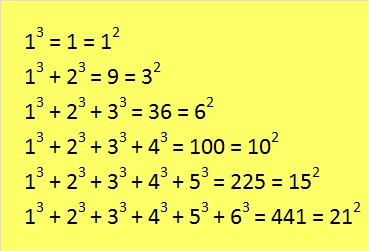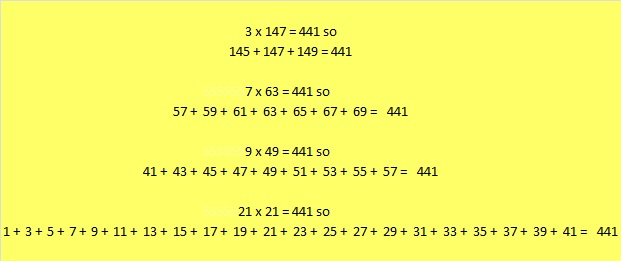# 441 Consecutive Numbers

The first 6 triangular numbers are

• 1 = 1
• 1 + 2 = 3
• 1 + 2 + 3 = 6
• 1 + 2 + 3 + 4 = 10
• 1 + 2 + 3 + 4 + 5 = 15
• 1 + 2 + 3 + 4 + 5 + 6 = 21

For millennia mathematicians have thought triangular numbers were quite interesting. 21 is a triangular number and 21 x 21 = 441 which makes 441 interesting, too. But wait, there’s something else that is very interesting about triangular numbers:It is amazing that when we begin with 1 cube, the sum of n consecutive cubes equals the nth triangular number squared every time!

What  follows is less amazing, but very practical. We can add consecutive numbers several ways to get 441. To find those ways we need to know the factors of 441.

• 441 is a composite number.
• Prime factorization: 441 = 3 x 3 x 7 x 7, which can be written 441 = (3^2) x (7^2)
• The exponents in the prime factorization are 2 and 2. Adding one to each and multiplying we get (2 + 1)(2 + 1) = 3 x 3 = 9. Therefore 441 has exactly 9 factors.
• Factors of 441: 1, 3, 7, 9, 21, 49, 63, 147, 441
• Factor pairs: 441 = 1 x 441, 3 x 147, 7 x 63, 9 x 49, or 21 x 21
• 441 is a perfect square. √441 = 21Because all of the factors for 441 are odd numbers, it is so easy to find consecutive numbers whose sum equal 441. Check out all of these:If we allowed negative numbers in the list of consecutive numbers we could also see that 441 equals the sum of 147 consecutive numbers that are centered around the number 3, and 49 consecutive numbers that are centered around the number 7, and 63 consecutive numbers that are centered around the number 9. All of those sums would be quite long.

Here are some more reasonable-length sums using only consecutive ODD numbers.That last sum reminds us that we always get n squared when we begin with one and add n consecutive odd numbers together.